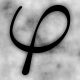## Quick Puzzles

Can you cut a cake in 8 pieces with just three slices?The following multiplication problem uses each number 0-9 only once. What are the missing numbers for *?

2** X *4 = **0**

What is the difference between half a dozen dozen and six dozen dozen? (It’s not nothing).

The following equation is wrong: 101 – 102 = 1.
Move one numeral to make it correct.

Two girls wish to cross a river. The only way to get to the other side is by boat, but that boat can only take one girl at a time. The boat cannot return on its own, there are no ropes or similar tricks, yet both girls manage to cross using the boat. How?

** Note: Some posts on Math-Fail are user-submitted and NOT verified by the admin of the site before publication. If you find this post to be distasteful, non-math related, ?or something worse?, then definitely leave a comment letting me know. Thanks very much! Mike **(3.00 from 3 votes)Loading...

## 10 Comments.

1.Ax

b) 5.5 dozen dozen
c) Move the 2 to be a power so it reads 101-10^2=110

2.Or

It just might be that my English isn’t very well, but I fail to see why (b) is a puzzle at all.
Isn’t “half a dozen dozen” just 12*12/2=72, and “six dozen dozen” just 6*12*12=864, and so the difference is 792?
Am I missing something here? Why would we need a hint that “It’s not nothing”? How could they be equal?00

3.Ax

a) 297 X 54 = 16038
There may be other solutions, this was the first I found and I was brute forcing a bit by hand so I stopped.10

4.Ax

It’s more of an English puzzle than a math puzzle. Native English speakers who have the phrase “half a dozen” hard wired in their mind to mean “six” usually have trouble parsing the difference in the two values with a casual reading.00

5.Maggie

(d) first one girl takes the boat across the river, and comes back. Then the second girl gets in the boat, crosses the river, and comes back. doesn’t say they both have to end up on the other side of the river at the same time!00

6.vsego

Nope, it’s the only solution:
perl -MAlgorithm::Permute -e ‘@x=(1,3,5..9); Algorithm::Permute::permute { print “@x\n” if “2\$x\$x” * “\$x4” == “\$x\$x0\$x\$x”;} @x;’
9 7 5 1 6 3 800

7.Actorclavilis

D) One girl is on one side, the other on the other. One crosses, then the other.00

8.djb

I didnt come up with the “101-10^2=1” solution from
above, but I did come up with the following..
101 = 102 – 1
OK, it doesnt move a ‘numeral’, it only moves a
‘line segment’, but it was all I came up with.00

9.Bryan Lee Williams

The following equation is wrong: 101 – 102 = 1.
Move one numeral to make it correct.
101 – 10 = 21.
Convert from base 3 to base 10, 10 – 3 = 700

10.Edgy

101 – 102 = 1
Remove 1 numeral to make it correct…
101 – 10 = 1
Its a binary equation.01